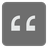Sum by Type
Elementary+
English

You have a list. Each value from that list can be either a string or an integer. Your task here is to return two values. The first one is a concatenation of all strings from the given list. The second one is a sum of all integers from the given list.

Input: An array of strings ans integers

Output: A list or tuple

Example:

```sum_by_types([]) == ['', 0]
sum_by_types([1, 2, 3]) == ['', 6]
sum_by_types(['1', 2, 3]) == ['1', 5]
sum_by_types(['1', '2', 3]) == ['12', 3]
sum_by_types(['1', '2', '3']) == ['123', 0]
sum_by_types(['size', 12, 'in', 45, 0]) == ['sizein', 57]
```

How it’s used: (math is used everywhere)

Precondition: both given ints should be between -1000 and 1000